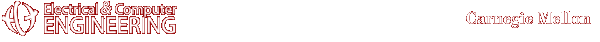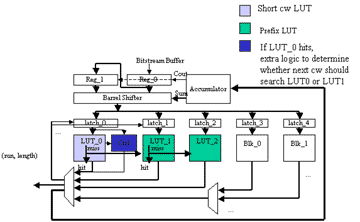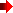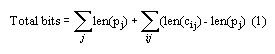Advanced Multimedia Processing Lab -- Projects -- Low power VLC Decoder#Edward Lin eclin@andrew.cmu.eduTop of this page

 Motivation and Goal

In the past, power efficiency in multimedia applications have largely been overlooked for high throughput designs. But with the rise in demand for portable multimedia devices and the high cost of cooling systems, power has become an important element in multimedia applications. One aspect of multimedia application systems is variable length decoding, which is used for decompression. By designing a custom hardware to decode the bitstream, a low power design can be realized.Top of this page

Optimal Prefixing

Because there are many different possible combinations of prefixes, an algorithm must be implemented to efficiently determine the best set of prefixes that would reduce the memory space optimally in large variable length tables. Useful prefixes—considering both breadth and depth-reduce redundancies in the codebook. The effectiveness of a prefixing scheme can be measured by the amount of bits saved by the removing unnecessary bit storage.

The proposed scheme uses optimal prefixing to partition VLC table lookup. Using the following example, we examine the ability to reduce the size of the overall codebook area by exhaustive search.Table 1: (a)Codebook with circled prefixes (b) Prefix comparison

For example, given a 5-entry variable length codebook as shown in Table 1. Let each codeword entry be denoted as cij with ij being codeword i with prefix entry j, while each prefix is expressed as pj and its length as len(pj).

Consider the possible set of prefix values for the codebook as shown in Table 1a. If the cost of each stored bit is 1, the total codebook cost is 17 without prefixing, calculated using (1).In Table 1b, Prefix 1 is unique and introduces a reduction in the total size of the table by 1 bit through condensing the preceding bit of the first 2 codewords. There are 3 ways to partition the rest of the codebook—with prefixes 0, 00, or 000. Prefix 0, 00, and 000 has reduction of 2, 2, and 3 bits, thus prefix 000 is chosen. The total cost for the memory given both prefix 1 and 000 is 12. The optimal memory efficient decoding problem is solved when the minimum number of bits needed to be stored after prefixing is found.

Parallel decoding

Fine grain table partitioning had been preposed before which reduce the power of the design according to codeword length. Given the previous low power design, control logic can be added in the first small table lookup to speed up decode with minimal overhead in area. When the short-codeword LUT is searched, the next few bits of the stream can be used to decode the next codeword, increasing throughput with little overhead. Since short codewords have the property of a 1 in the first few bits, an OR of the first few bits of the next codeword can determine which LUT to begin searching (Figure 1).Figure 1. Proposed Low power variable length decoderTop of this page

Any suggestions or comments are welcome. Please send them to Edward LinTop of this page